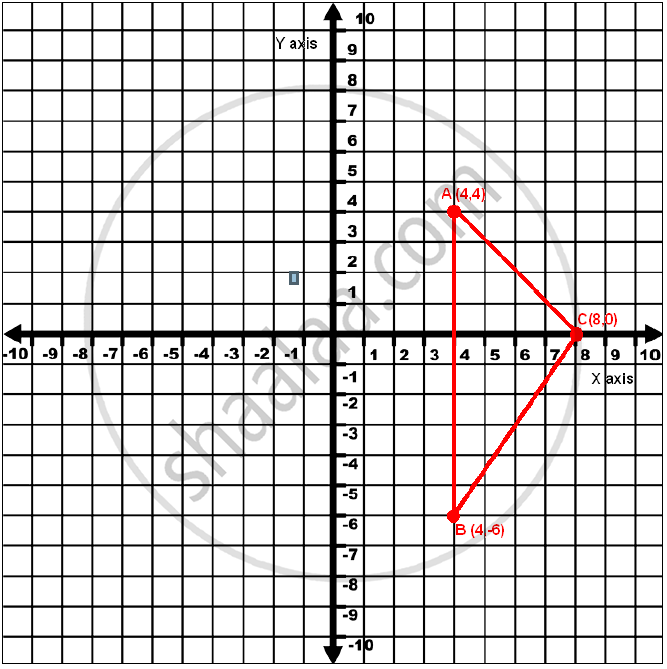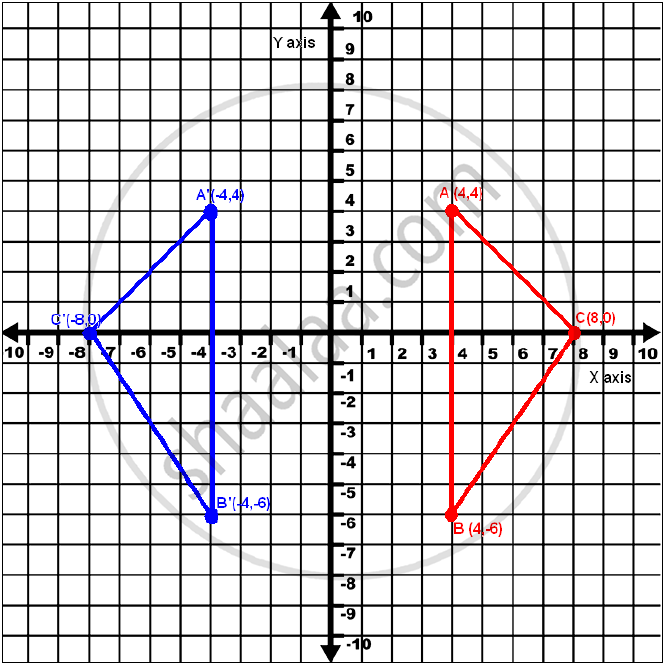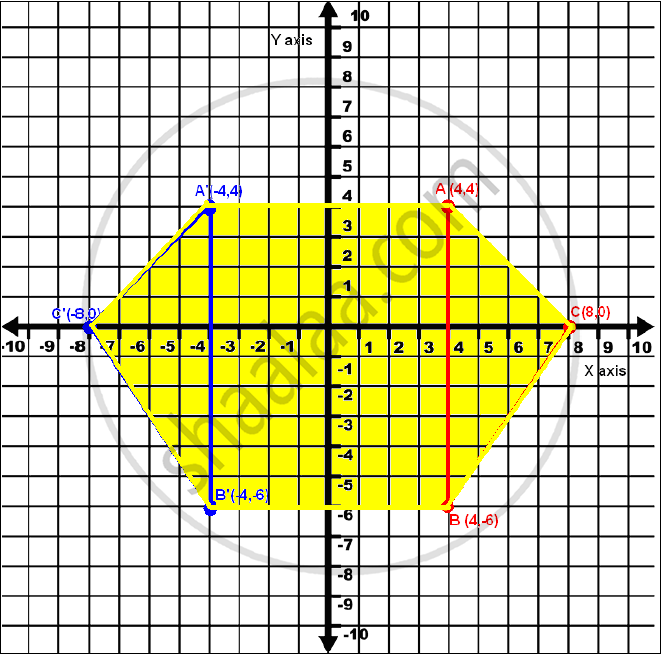Share

# Use a Graph Paper to Answer the Following Questions (Take 1 Cm = 1 Unit on Both Axes) 1) Plot A(4,4), B(4,-6) and C(8,0), the Vertices of a Triangle Abc. 2) Reflect Abc on the Y-axis and Name It A’B’C’. 3) Write the Coordinates of the Images A’, B’ and C’. 4) Give a Geometrical Name for the Figure Aa’ C’ B’ Bc. - ICSE Class 10 - Mathematics

#### Question

Use a graph paper to answer the following questions (Take 1 cm = 1 unit on both axes)

1) Plot A(4,4), B(4,-6) and C(8,0), the vertices of a triangle ABC.

2) Reflect ABC on the y-axis and name it A’B’C’.

3) Write the coordinates of the images A’, B’ and C’.

4) Give a geometrical name for the figure AA’ C’ B’ BC.

5) Identify the line of symmetry of AA’ C’ BC’.

#### Solution

1) The points A(4, 4), B(4, -6) and C(8, 0) are plotted on the coordinate plane as follows:2) To reflect the points ABC across Y axis we will keep y coordinate as it is and negate the x coordinate.The reflected image of the triangle is shown in blue.

3) The coordinates of point A’, B’, C’ are (-4, 4), (-4,-6) and (-8, 0) respectively.5) The line of symmetry of AA’C’B’BC would be the y axis.

Is there an error in this question or solution?

#### APPEARS IN

2010-2011 (March) (with solutions)
Question 6.1 | 4.00 marks

#### Video TutorialsVIEW ALL 

Solution Use a Graph Paper to Answer the Following Questions (Take 1 Cm = 1 Unit on Both Axes) 1) Plot A(4,4), B(4,-6) and C(8,0), the Vertices of a Triangle Abc. 2) Reflect Abc on the Y-axis and Name It A’B’C’. 3) Write the Coordinates of the Images A’, B’ and C’. 4) Give a Geometrical Name for the Figure Aa’ C’ B’ Bc. Concept: Lines of Symmetry.
S